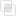Python中numpy求函数的导数实现方法

numpy2021-03-24 16:28:1511413浏览 · 0收藏 · 0评论• 可以使用Finite Differences

• 可以使用Automatic Derivatives

• 可以使用Symbolic Differentiation

• 可以手动计算导数。

```In : from sympy import *
In : import numpy as np
In : x = Symbol('x')
In : y = x**2 + 1
In : yprime = y.diff(x)
In : yprime
Out: 2⋅x

In : f = lambdify(x, yprime, 'numpy')
In : f(np.ones(5))
Out: [ 2.  2.  2.  2.  2.]```

（推荐操作系统：windows7系统、Python 3.9.1，DELL G3电脑。）Python中如何使用numpy.getmask()函数Python中的numpy怎样实现结构体Python中numpy数组如何添加和删除元素Python中numpy怎样按行或列提取矩阵Python基础：numpy中空值怎样设置Python实用之numpy中空数组的创建Python之numpy中mask选取子集Python基础：numpy中vstack和hstack函数Python中如何用numpy解决梯度下降最小值Python基础：numpy中的常见函数有哪些python学习网